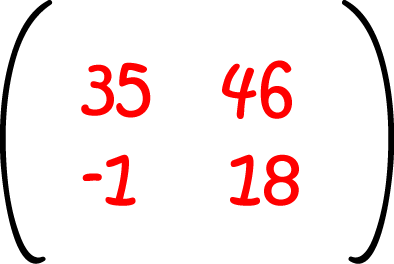# Definition of MatrixA matrix is an array of numbers arranged in rows and columns.

The plural of matrix is "matrices".

Matrices can be added, subtracted, multiplied, multiplied by scalars and inverted. You can even take functions of matrices.

Matrices provide a useful tool for solving systems of linear equations.

The field of "Linear Algebra" deals with matrices and their properties.

### Description

The aim of this dictionary is to provide definitions to common mathematical terms. Students learn a new math skill every week at school, sometimes just before they start a new skill, if they want to look at what a specific term means, this is where this dictionary will become handy and a go-to guide for a student.

### Audience

Year 1 to Year 12 students

### Learning Objectives

Learn common math terms starting with letter M

Author: Subject Coach
You must be logged in as Student to ask a Question.